# 局部最小值和全局最小值¶

f(x) = x*cos(\pi*x)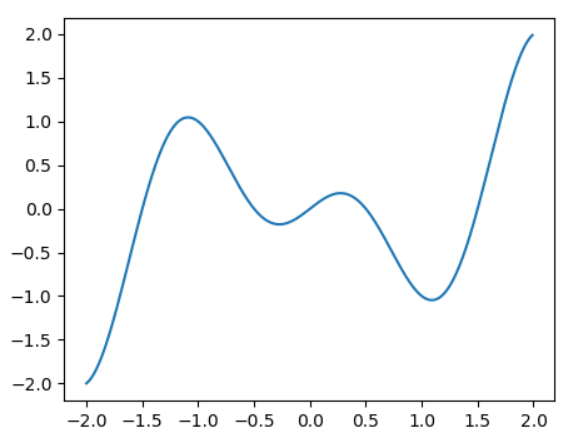import matplotlib.pyplot as plt
import numpy as np

x = np.arange(-2, 2, 0.01)
print(x)
f = x*np.cos(np.pi*x)
plt.plot(x, f)
plt.show()


# 鞍点¶

f(x) = x^3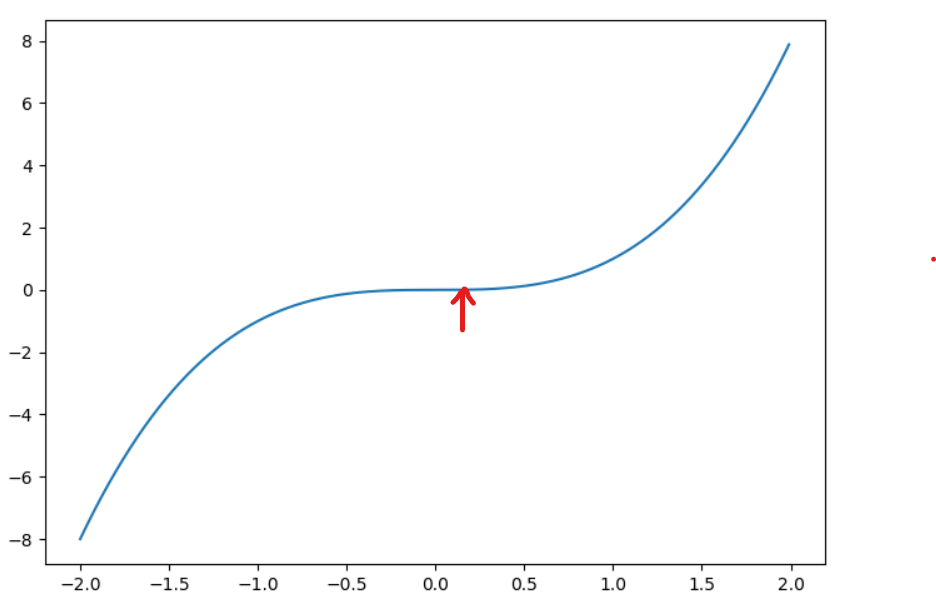# 梯度下降为什么有效¶

f(x+\epsilon) = f(x) + \epsilon*f(x)'

f(x)'代表x处的梯度

f(x-n*f(x)’) = f(x)-n*f(x)'^2

f(x - n*f(x)') < f(x)

f(x) = x*cos(\pi*x)

import matplotlib.pyplot as plt
import numpy as np

x = 0.5
results = [x]
for i in range(5):
# 函数x*np.cos(np.pi*x)的导数为np.cos(np.pi * x) - x * np.sin(np.pi * x)
x -= eta * (np.cos(np.pi * x) - x * np.sin(np.pi * x))
results.append(x)
return results

def fx(arr):
ans = []
for num in arr:
ans.append(num * np.cos(np.pi * num))
return ans

def plot():
x = np.arange(-2, 2, 0.01)
y = x * np.cos(np.pi * x)
res_y = fx(res)
plt.plot(x, y)
plt.plot(res, res_y, '-o')
plt.show()

plot()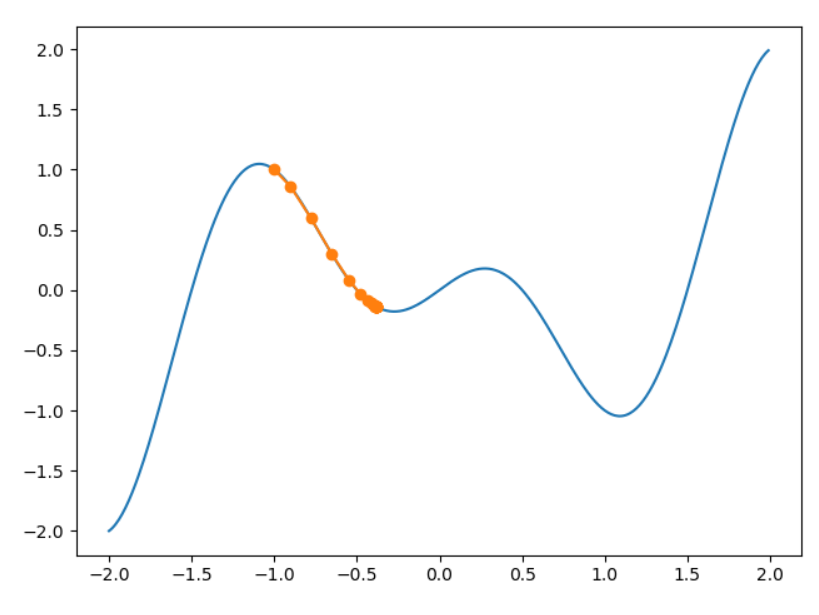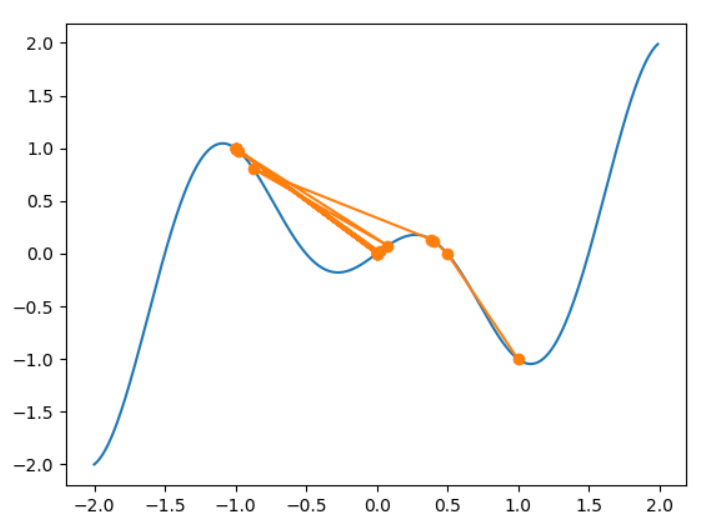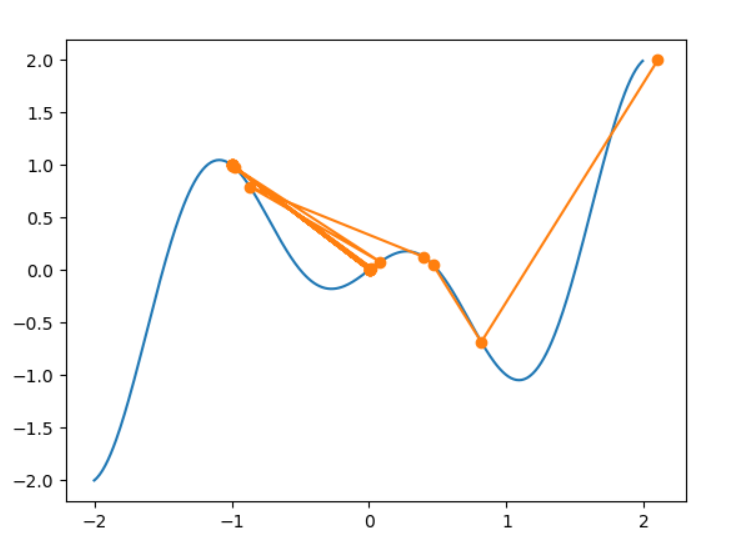# 动量法Momentum¶

v_t = \gamma*v_{t-1} + \eta_t*g_t \\ x_t = x_{t-1} - v_t

## 指数移动平均¶

y{t} = (1-\gamma)*x_t + \gamma*y_{t-1}

y_t = (1-\gamma)*x_t + (1-\gamma)*\gamma*x_{t-1} + \gamma^2*y_{t-2}\\ y_t = (1-\gamma)*x_t + (1-\gamma)*\gamma*x_{t-1} + (1-\gamma)*\gamma^2*x_{t-2} + \gamma^3*y_{t-2}\\ 令 n = \frac{1} {(1-\gamma)}\\ 则 (1-1/n)^n = \gamma^{1/(1-\gamma)} 这里直接是变量替换\\ 而我们由高数的常见的极限公式可得\\ lim_{n \to \infty}(1- \frac{1}{n})^n = \frac{1}{e} = 0.37 \\ 而\gamma \rightarrow 1，则 n \rightarrow \infty \\ 举个例子，取\gamma = 0.9，则根据前面的极限公式 0.9^{10} \rightarrow 0.37 \\ 如果我们把0.37看作是个很小的数，那我们可以忽略了更高阶的系数，比如0.9^{11}, 0.9^{12}等等\\ 换句话说，当\gamma = 0.9，我们只关注近十个阶数的系数

v_t = (1-\gamma)*(\frac{\eta}{1-\gamma}*g_t) + \gamma*v_{t-1}

(\frac{\eta}{1-\gamma}*g_t)

s_t = s_{t-1} + g_t \odot g_t \\ x_t = x_{t-1} + \frac{\eta} {\sqrt{s_t + \epsilon}} \odot g_t \\ \epsilon 是一个很小的值，防止除0

# RMSProp算法¶

s_t = \gamma*s_{t-1} +(1-\gamma) * g_t \odot g_t \\ x_t = x_{t-1} + \frac{\eta} {\sqrt{s_t + \epsilon}} \odot g_t \\ \epsilon 是一个很小的值，防止除0

v_t = \beta_1*v_{t-1} + (1-\beta_1)*g_t \\ s_t = \beta_2*s_{t-1} + (1-\beta_2)* g_t \odot g_t

v_t = \frac {v_t}{1-\beta1} \\ s_t = \frac {s_t}{1-\beta2} \\

g_t' = \frac{\eta*v_t}{\sqrt{s_t}+\epsilon} 这里\epsilon是一个很小的数 \\ x_t = x_{t-1} - g_t'